# Why is 1441 a Star?

1441 is the 16th star number. If you look at the tiny squares that make up each triangle in the star, you might notice that each of those triangles is made with 15 rows of squares. Thus each triangle represents the 15th triangular number.A six-pointed star is really just a 12-sided figure better known as a dodecagon.

Since I recently wrote that figurate numbers can be found using triangular numbers, I wondered if centered figurate numbers can make the same claim. Yes, they can!

Centered Figurate Numbers are also called Centered Polygonal Numbers and can be easily calculated from the triangular numbers.Here are some more facts about the number 1441:

• 1441 is a composite number.
• Prime factorization: 1441 = 11 × 131
• 1441 has no exponents greater than 1 in its prime factorization, so √1441 cannot be simplified.
• The exponents in the prime factorization are 1, and 1. Adding one to each exponent and multiplying we get (1 + 1)(1 + 1) = 2 × 2 = 4. Therefore 1441 has exactly 4 factors.
• The factors of 1441 are outlined with their factor pair partners in the graphic below.1441 is a palindrome with an even number of digits so it is divisible by 11.

1441 is also a palindrome in some other bases, namely, base 6, base 30, base 32, and base 36.

# Taking the Mystery out of 1440

The number 1440 has thirty-six factors. That’s a lot! The only number smaller than it with that many factors is 1260. Why do they have so many?

Would solving this Mystery Level puzzle be an easier mystery to solve?Print the puzzles or type the solution in this excel file: 10 Factors 1432-1442

• 1440 is a composite number.
• Prime factorization: 1440 = 2 × 2 × 2 × 2 × 2 × 3 × 3 × 5, which can be written 1440 = 2⁵ × 3² × 5
• 1440 has at least one exponent greater than 1 in its prime factorization so √1440 can be simplified. Taking the factor pair from the factor pair table below with the largest square number factor, we get √1440 = (√144)(√10) = 12√10
• The exponents in the prime factorization are 5,2, and 1. Adding one to each exponent and multiplying we get (5 + 1)(2 + 1)(1 + 1) = 6 × 3 × 2 = 36. Therefore 1440 has exactly 36 factors.
• The factors of 1440 are outlined with their factor pair partners in the graphic below.There you have it. The exponents of the prime factorization determine the number of factors a number has, and (5 + 1)(2 + 1)(1 + 1) = 6 × 3 × 2 = 36. How do we find its prime factorization? We can divide it over and over by the smallest prime number factor until we make an easy-to-read and very delicious cake!Or you could make a factor tree such as the one below. Then rake up the prime number factors afterward and hope that you see them all:I prefer cake to yard work any day!

As I mentioned before, 1440 is NOT the smallest number with 36 factors:
Because (2 + 1)(2 + 1)(1 + 1)(1 + 1) = 3 × 3 × 2 × 2 = 36 also, we get
2²× 3²× 5 × 7 = 1260, which just happens to be smaller than 1440, so 1260 gets the smallest-number-with-36-factors prize. 1440 is the SECOND smallest number with 36 factors.

1440 is the sum of the interior angles of a decagon. Why?
Because 180(10-2) = 180(8) = 1440
So what? 1260 is the sum of the interior angles of a nonagon.

1440 is the hypotenuse of only one Pythagorean triple:
864-1152-1440 which is (3-4-5) times 288
Yeah? 1260 is the hypotenuse of only one Pythagorean triple as well.

Look, 1440 is not second best because it has this one other claim to fame:
From Stetson.edu we learn that 1440 deserves a lot of exclamation points since
1440 = 2!3!5!

There you have it. We’ve taken the mystery out of the number 1440, AND it is a fabulous number!

# 1439 and Level 6

You know the factors of 49, but to complete this puzzle, you will also have to determine the answers to a few questions:

Will the common factor of 24 and 32 be 4 or 8?
Will the common factor of 20 and 40 be 4, 5, or 10?
Will the common factor of 27 and 9 be 3 or 9?

Don’t guess and check! Look at the other clues, and some of the possibilities will be eliminated*. Once you’ve found the next clue to use, continue using logic until the whole puzzle is completed.Print the puzzles or type the solution in this excel file: 10 Factors 1432-1442

• 1439 is a prime number.
• Prime factorization: 1439 is prime.
• 1439 has no exponents greater than 1 in its prime factorization, so √1439 cannot be simplified.
• The exponent in the prime factorization is 1. Adding one to that exponent we get (1 + 1) = 2. Therefore 1439 has exactly 2 factors.
• The factors of 1439 are outlined with their factor pair partners in the graphic below.How do we know that 1439 is a prime number? If 1439 were not a prime number, then it would be divisible by at least one prime number less than or equal to √1439. Since 1439 cannot be divided evenly by 2, 3, 5, 7, 11, 13, 17, 19, 23, 29 31, or 37, we know that 1439 is a prime number.

* Did you notice that either one of the 24’s or 6 must use a 3 because only two 6s are allowed to be used as factors? What does that tell you about the common factor of 27 and 9?

# 1438 and Level 5

You can solve this puzzle by using logic and multiplication/division facts. The unique solution requires all the numbers from 1 to 10 in both the first column and the top row. Can you solve it?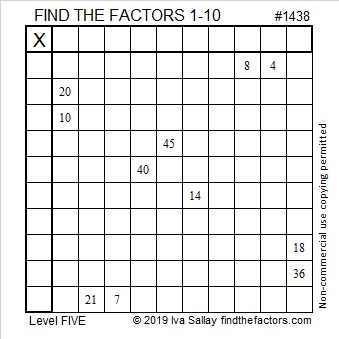Print the puzzles or type the solution in this excel file:  10 Factors 1432-1442

Today’s puzzle was given the number 1438. Here are a few facts about that number:

• 1438 is a composite number.
• Prime factorization: 1438 = 2 × 719
• 1438 has no exponents greater than 1 in its prime factorization, so √1438 cannot be simplified.
• The exponents in the prime factorization are 1, and 1. Adding one to each exponent and multiplying we get (1 + 1)(1 + 1) = 2 × 2 = 4. Therefore 1438 has exactly 4 factors.
• The factors of 1438 are outlined with their factor pair partners in the graphic below.1438 is the sum of four consecutive numbers:
358  + 359 + 360 + 361 = 1438

# 1437 Belt Buckle Mystery

I have childhood memories of preparing for Thanksgiving at school by making pilgrim hats, pilgrim shirts, or pilgrim shoes out of construction paper. Each of those clothing items had a distinguished buckle. Why? That buckle’s popularity was a mystery to me until I read an article by Ken Jennings titled The Debunker: What Did Pilgrim Hats Really Look Like?

This mystery level puzzle has a buckle, too. Perhaps it can help debunk the idea that math is no fun while it helps you learn about factors or reinforces your memory of the multiplication table.Print the puzzles or type the solution in this excel file:  10 Factors 1432-1442

The puzzle number is 1437. Here are a few facts about that number:

• 1437 is a composite number.
• Prime factorization: 1437 = 3 × 479
• 1437 has no exponents greater than 1 in its prime factorization, so √1437 cannot be simplified.
• The exponents in the prime factorization are 1, and 1. Adding one to each exponent and multiplying we get (1 + 1)(1 + 1) = 2 × 2 = 4. Therefore 1437 has exactly 4 factors.
• The factors of 1437 are outlined with their factor pair partners in the graphic below.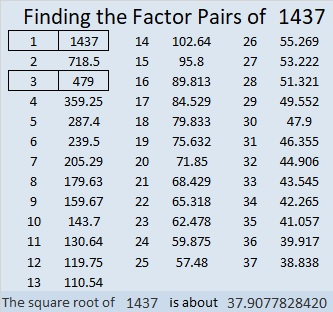1437 is the difference of two squares in two different ways:
719² – 718² = 1437
241² – 238² = 1437

# 1436 This Turkey Is Ready

If this year you find yourself needing a bigger turkey, then this one fits that description. This turkey uses math facts from a 14 × 14 multiplication table.

Adding prime number 13 doesn’t cause any problems. You hopefully will recognize that 39 and 119 are both multiples of 13.

However, adding 14 to the puzzle might make you want to call “fowl” because clues 28, 56, 70, and 84 all have two sets of factor pairs where both factors are 14 or less. Can you figure out which clues use 7 and which use 14?

Like always, there is only one solution.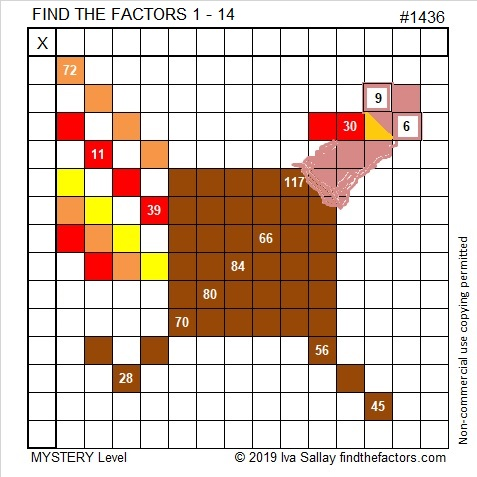Print the puzzles or type the solution in this excel file:  10 Factors 1432-1442

I enjoyed this tweet from Robin Schwartz. Spoiler alert: If you click on the link, you will see the solution.

That was puzzle number 1436. Here are a few facts about that number:

• 1436 is a composite number.
• Prime factorization: 1436 = 2 × 2 × 359, which can be written 1436 = 2² × 359
• 1436 has at least one exponent greater than 1 in its prime factorization so √1436 can be simplified. Taking the factor pair from the factor pair table below with the largest square number factor, we get √1436 = (√4)(√359) = 2√359
• The exponents in the prime factorization are 2 and 1. Adding one to each exponent and multiplying we get (2 + 1)(1 + 1) = 3 × 2 = 6. Therefore 1436 has exactly 6 factors.
• The factors of 1436 are outlined with their factor pair partners in the graphic below.1436 is the difference of two squares:
360² – 358² = 1436

# 1435 is the 23rd Friedman Number

41 ×35 = 1435. Since the same digits are used on both sides of the equal sign that makes 1435 the 23rd Friedman number.

Since it’s good for puzzles to have a puzzle number to distinguish them from each other, I’ve given this one the number 1435. Can you solve it?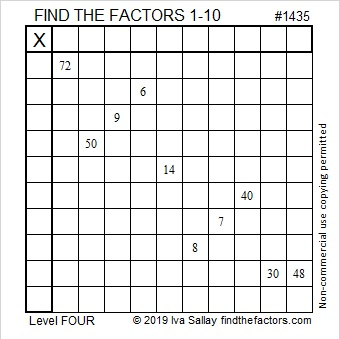Print the puzzles or type the solution in this excel file:  10 Factors 1432-1442

Here are a few more facts about the number 1435:

• 1435 is a composite number.
• Prime factorization: 1435 = 5 × 7 × 41
• 1435 has no exponents greater than 1 in its prime factorization, so √1435 cannot be simplified.
• The exponents in the prime factorization are 1, 1, and 1. Adding one to each exponent and multiplying we get (1 + 1)(1 + 1)(1 + 1) = 2 × 2 × 2 = 8. Therefore 1435 has exactly 8 factors.
• The factors of 1435 are outlined with their factor pair partners in the graphic below.1435 is also the hypotenuse of FOUR Pythagorean triples:
315-1400-1435 which is 35 times (9-40-41)
588-1309-1435 which is 7 times (84-187-205)
861-1148-1435 which is (3-4-5) times 287
931-1092-1435 which is 7 times (133-156-205)

# 1434 and Level 3

If you know the common factors of 14 and 8, then you will have an excellent start to solve this puzzle. Once you place the factors of 18 and 8 in their proper places, just work down the puzzle row by row until all the factors from 1 to 10 are found.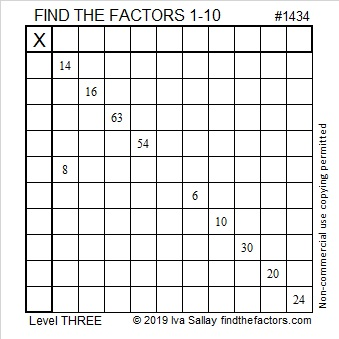Print the puzzles or type the solution in this excel file:  10 Factors 1432-1442

Now I’ll share some facts about the puzzle number, 1434:

• 1434 is a composite number.
• Prime factorization: 1434 = 2 × 3 × 239
• 1434 has no exponents greater than 1 in its prime factorization, so √1434 cannot be simplified.
• The exponents in the prime factorization are 1, 1, and 1. Adding one to each exponent and multiplying we get (1 + 1)(1 + 1)(1 + 1) = 2 × 2 × 2 = 8. Therefore 1434 has exactly 8 factors.
• The factors of 1434 are outlined with their factor pair partners in the graphic below.1434 is 234 in BASE 26 because
2(26²) + 3(26¹) + 4(26º) = 1434

# 1433 and Level 2

There is only one way to arrange the numbers from 1 to 10 in both the first column and the top row to make this puzzle function like a multiplication table. Can you find that one way?Print the puzzles or type the solution in this excel file:  10 Factors 1432-1442

The puzzle number is 1433. Here are some facts about that number:

• 1433 is a prime number.
• Prime factorization: 1433 is prime.
• 1433 has no exponents greater than 1 in its prime factorization, so √1433 cannot be simplified.
• The exponent in the prime factorization is 1. Adding one to that exponent we get (1 + 1) = 2. Therefore 1433 has exactly 2 factors.
• The factors of 1433 are outlined with their factor pair partners in the graphic below.

How do we know that 1433 is a prime number? If 1433 were not a prime number, then it would be divisible by at least one prime number less than or equal to √1433. Since 1433 cannot be divided evenly by 2, 3, 5, 7, 11, 13, 17, 19, 23, 29 31, or 37, we know that 1433 is a prime number.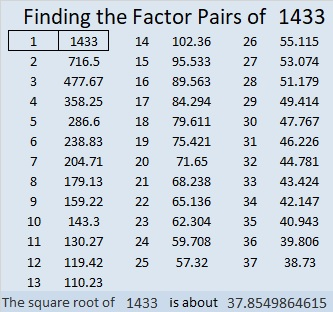1433 is the sum of two squares:
37² + 8² = 1433

1433 is the hypotenuse of a Pythagorean triple:
592-1305-1433 calculated from 2(37)(8), 37² – 8², 37² + 8²

Here’s another way we know that 1433 is a prime number: Since its last two digits divided by 4 leave a remainder of 1, and 37² + 8² = 1433 with 37 and 8 having no common prime factors, 1433 will be prime unless it is divisible by a prime number Pythagorean triple hypotenuse less than or equal to √1433. Since 1433 is not divisible by 5, 13, 17, 29, or 37, we know that 1433 is a prime number.

# 1432 and Level 1

If you can count by tens, then you can solve this Level 1 puzzle. I dare you to prove me wrong!Print the puzzles or type the solution in this excel file:  10 Factors 1432-1442

Here is some information about the puzzle number, 1432:

• 1432 is a composite number.
• Prime factorization: 1432 = 2 × 2 × 2 × 179, which can be written 1432 = 2³ × 179
• 1432 has at least one exponent greater than 1 in its prime factorization so √1432 can be simplified. Taking the factor pair from the factor pair table below with the largest square number factor, we get √1432 = (√4)(√358) = 2√358
• The exponents in the prime factorization are 3 and 1. Adding one to each exponent and multiplying we get (3 + 1)(1 + 1) = 4 × 2 = 8. Therefore 1432 has exactly 8 factors.
• The factors of 1432 are outlined with their factor pair partners in the graphic below.1432 is 21212 in BASE 5 because
2(5⁴) + 1(5³) + 2(5²) + 1(5¹) + 2(5⁰) = 1432